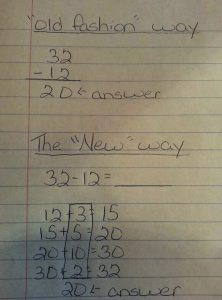# Calculation was the price we used to have to pay to do mathematics

Keith Devlin in Devlin’s Angle:Ever since mathematics got properly underway around 3,000 years ago, there was only one way to achieve access to the field. You had to spend many years developing a fairly extensive calculation skillset. In the first instance, to pass the graduation and entrance examinations to gain initial access to the field. Then, once accepted into the world of mathematics, calculation of one kind or another was what all mathematicians spent the bulk of their mathematical time doing. Arguably, for most of mathematics history, the subject really was, to a large extent, primarily about calculation of one form or another. Newton, Leibniz, Bernoulli (any of them), Fermat, Euler, Riemann, Gauss, and the other greats of times past, were all superb masters of calculation. (We should also include Boole, since his famous Boolean algebra is also a calculation system.)

But whereas most laypersons seem to think that calculation is all there is to mathematics, surely none of the greats did. Calculation was an important tool (more accurately, a set of tools) you needed to do mathematics, they must have realized, but the essence of mathematics is much more, a plateau of knowledge that transcends all the calculation techniques.

More here.